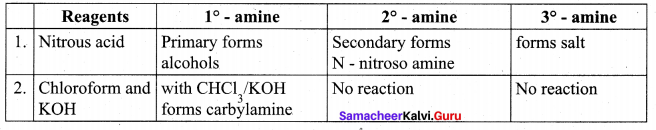# Tamil Nadu 12th Chemistry Model Question Paper 4 English Medium

Students can Download Tamil Nadu 12th Chemistry Model Question Paper 4 English Medium Pdf, Tamil Nadu 12th Chemistry Model Question Papers helps you to revise the complete Tamilnadu State Board New Syllabus and score more marks in your examinations.

## TN State Board 12th Chemistry Model Question Paper 4 English Medium

Instructions:

1. The question paper comprises of four parts
2. You are to attempt all the parts. An internal choice of questions is provided wherever: applicable
3. All questions of Part I, II, III, and IV are to be attempted separately
4. Question numbers 1 to 15 in Part I are Multiple choice Questions of one mark each.  These are to be answered by choosing the most suitable answer from the given four alternatives and writing the option code and the corresponding answer
5. Question numbers 16 to 24 in Part II are two-mark questions. These are lo be answered in about one or two sentences.
6. Question numbers 25 to 33 in Part III are three-mark questions. These are lo be answered in about three to five short sentences.
7. Question numbers 34 to 38 in Part IV are five-mark questions. These are lo be answered in detail. Draw diagrams wherever necessary.

Time: 3 Hours
Maximum Marks: 70

Part-I

Answer all the questions. Choose the correct answer. [15 × 1 = 15]

Question 1.
The metal oxide which cannot be reduced to metal by carbon is ………………….
(a) PbO
(b) Al2O3
(C) ZnO
(d) FeO
(b) Al2O3

Question 2.
Compounds used as an eye lotion .
(a) H3BO3
(b) HBO2
(C) H2B4O7
(d) B2O3
(a) H3BO3

Question 3.
Which one of the following orders is correct for the bond dissociation enthalpy of halogen molecules?
(a) Br 2> I2 > F2 > Cl2
(b) F2 > Cl2 > Br2 > I2
(c) I2 > Br2 > Cl2 > F2
(d) Cl2 > Br2 > F2 > I2
(d) Cl2 > Br2 > F2 > I2Question 4.
Consider the following statements.
(i) The melting point decreases from Scandium to Vanadium in 3d series.
(ii) In 3d transition series, atomic radius decreases from Sc to V and upto copper atomic radius nearly remains the same.
(iii) As we move down in 3d transition series, atomic radius increases.
Which of the above statements is/are incorrect?
(a) i only
(b) ii only
(c) iii only
(d) i, ii and iii
(a) i only

Question 5.
Which complex is used as an antitumor drug in cancer treatment?
(a) Ca – EDTA chelate
(b) EDTA
(c) Ti Cl4 + Al(C2H5)3
(d) Cis – Platin
(b) EDTA

Question 6.
The number of unit cells in 8 gm of an element X ( atomic mass 40) which crystallizes in bcc
pattern is (NA is the Avogadro number) ………..
(a) 6.023 x 1023
(b) 6.023 x 1022
(c) 60.23 x 1023
(d) $$\left(\frac{6.023 \times 10^{23}}{8 \times 40}\right)$$

Hint: In bcc unit cell, 2 atoms = 1 unit cell
Number of atoms in 8g of element is Number of moles = $$\frac{8 g}{40 g, \mathrm{mol}^{-1}}$$ = 0.2mol
1 mole contains 6.023 x 1023 atoms 40g,mol
0.2 mole contains 0.2 x 6.023 x 1023 atoms
$$\left(\frac{1 \text { unit cell }}{2 \text { atoms }}\right)$$ x 0.2 x 6.023 xio23 = 6.023 x 1022 unit cells

Question 7.
Among the following graphs showing variation of rate constant with temperature (T) for a reaction, the one that exhibits Arrhenius behavior over the entire temperature range is …………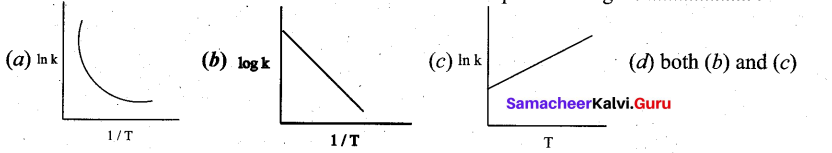Solution:
k = Ae-(Ea/RT)
lnk = $$\ln \mathrm{A}-\left(\frac{\mathrm{E}_{\mathrm{a}}}{\mathrm{R}}\right)\left(\frac{1}{\mathrm{T}}\right)$$
this equation is in the form of a straight line equation y = c + m x
a plot of lnk vs (1/T) is a straight line with negative slope.Question 8.
4Na+O2 → 2Na2O
Na2O + H2O → 2NaOH
In the given reaction, the oxide of sodium is …………..
(a) Acidic
(b) Basic
(c) Amphoteric
(d) Neutral
(b) Basic
Solution:
Na2O form NaOH so that it is basic oxide.

Question 9.
How many faradays of electricity are required for the following reaction to occur
MnO4 → Mn2+
(a) 5F
(b)3F
(e) 1F
(d) 7F
(a) 5F
Hint: 7MnO4 + 5e → Mn2+4H2O
5 moles of electrons i.e., 5F charge is required.

Question 10.
Match the following: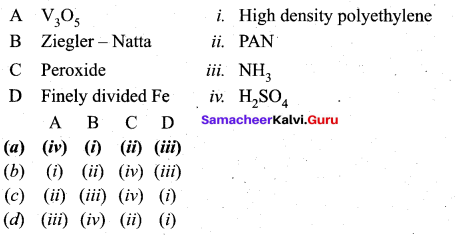(a) A – (iv), B – (i), C – (ii), D – (iii)Question 11.
Which of the following is not the product of dehydration of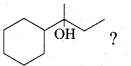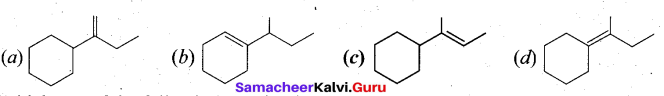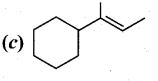Question 12.
Which one of the following reaction is an example of disproportionation reaction?
(a) Aldol condensation
(b) cannizaro reaction
(c) Benzoin condensation
(d) none of these
(b) cannizaro reaction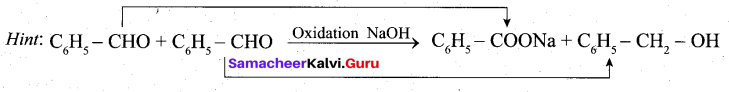Question 13.
The correct order of basic strength in the case of substituted ethyl amines is ………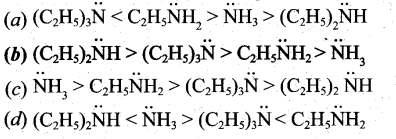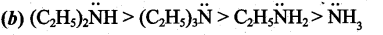Question 14.
Assertion: A solution of sucrose in water is dextrorotatory. But on hydrolysis in the presence
of little hydrochloric acid, it becomes levorotatory. –
Reason: Sucrose hydrolysis gives unequal amounts of glucose and fructose. As a result of this – change in sign of rotation is observed.
(a) If both accretion and reason are true and reason is the correct explanation of assertion
(b) If both assertion and reason are true but reason is not the correct explanation of assertion
(c) If assertion is true but reason is false
(d) if both assertion and reason are false
(a) If both accretion and reason are true and reason is the correct explanation of assertion

Question 15.
The correct structure of the drug paracetamol is ……….
Tamil Nadu 12th Chemistry Model Question Paper 4 English Medium – 9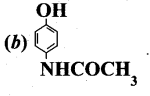Part – II

Answer any six questions. Question No. 20 is compulsory. [6 x 2 = 12]

Question 16.
What are the various steps involved in extraction of pure metals from their ores?
The extraction of pure metals from the concentrated ores is carried out in two steps:

• Conversion of the ore into oxides of the metal of interest.
• Reduction of the metal oxides to elemental metals.

Question 17.
Why white phosphorous is also known as yellow phosphorous?
The freshly prepared white phosphoms is colourless but becomes pale yellow due to formation of a layer of red phosphorus upon standing. Hence it is also known as yellow phosphoms.Question 18.
Give an example of coordination compound used in medicine.
Medical uses of coordination compounds : –

• Ca-EDTA chelate, is used in the treatment of lead and radioactive poisoning. That is for removing
• Cis-platin is used as an antitumor drug in cancer treatment.

Question 19.
Give the examples for a zero order reaction.
Examples for a zero order reaction:
(i) Photochemical reaction between H2 and Cl2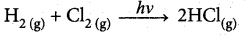(ii) Decomposition of N20 on hot platinum surface
N2 (g) ⇌ N2 + 1/2O2(g)

(iii) Iodination of acetone in acid medium is zero order with respect to iodine.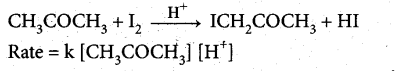Question 20.
Write the overall redox reaction which takes place in the galvanic cell,
Pt(s) | Fe2+(aq),Fe3+(aq) | MnO4(aq), H+(aq),Mn2+ (aq) | Pt(s)
At Anode half cell : 5Fe2+(aq) → 5Fe3+(aq) + 5e
At cathode half cell : MnO4(aq) + 8H+(aq) + 5e → Mn2+(aq) + 4H2O(l)
Overall redox reaction : 5Fe2+(aq) + MnO4(aq) + 8H+(aq)→ 5Fe3+(aq) + Mn2+(aq) + 4H2O(l)

Question 21.
In a coagulation experiment 10 mL of a colloid (X) is mixed with distilled water and 0.1M solution of an electrolyte AB so that the volume is 20 mL. It was found that all solutions containing more than 6.6 mL of AB coagulate with in 5 minutes. What is the flocculation values of AB for sol (X)?
A minimum of 6.6mL of AB is required to coagulate the sol.
The moles of AB in the sol is, $$\frac{6.6 \times 0.01}{20}$$ = 0.0033 moles
This means that a minimum of 0.033 moles or 0.0033 x 1000 = 3.3 milli moles are required
for coagulating one litre of sol.
Flocculation value of AB for X = 3.3Question 22.
Explain Rosenmund reduction.
Aldehydes can be prepared by the hydrogenation of acid chloride, in the presence of palladium supported by Barium sulphate. This reaction is called Rosenmund reduction.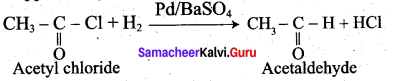In the above reaction, BaS04 act as a catalytic poison to palladium catalyst, so that aldehyde cannot be further reduced to alcohol.

Question 23.
‘What are the uses of aliphatic nitro compounds.
(i) Nitromethane is used as a fuel for cars.
(ii) Chloropicrin (CCl3NO2) is used as an insecticide
(iii) Nitroethane is used as a fuel additive and precursor to explosive and they are good solvents for polymers, cellulose ester, synthetic rubber and dyes etc.

Question 24.
Classify the following as linear, branched or cross linked polymers
(a) Bakelite
(b) Nylon
(c) polythene
(a) Bakelite – cross linked polymer
(b) Nylon – Linear polymer
(c) Polythene – Linear polymer

Part – III

Answer any six questions. Question No. 32 is compulsory. [6 x 3 = 18]

Question 25.
A hydride of 2nd period alkali metal (A) on reaction with compound of Boron (B) to give a reducing agent (C). identify A, B and C.
(i) A hydride of 2nd period alkali metal (A) is lithium hydride (LiH).
(ii) Lithium hydride (A) reacts with diborane (B) to give lithiumborohydride (C) which act as reducing agent.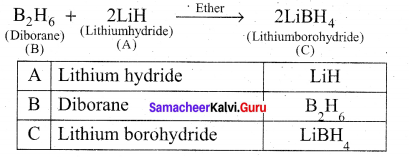Question 26.
Discuss the uses of phosphine.
Phosphine is used for producing smoke screen as it gives large smoke. In a ship, a pierced . container with a mixture of calcium carbide and calcium phosphide, liberates phosphine and acetylene when thrown into sea. The liberated phosphine catches fire and ignites acetylene. These burning gases serves as a signal to the approaching ships. This is known as Holmes signal.

Question 27.
Calculate the percentage efficiency of packing in case of body centered cubic crystal.
Packing efficiency : In body centered cubic arrangement the spheres are touching along the leading diagonal of the cube as shown in the figure.
In ∆ABC,
AC2 = AB2 + BC2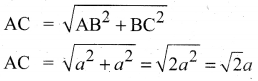In∆ACG,
AG2 = AC2 + CG2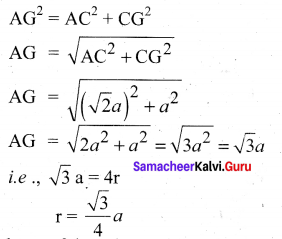∴ Volume of the sphere with radius ‘r’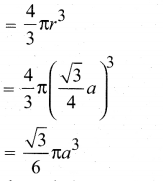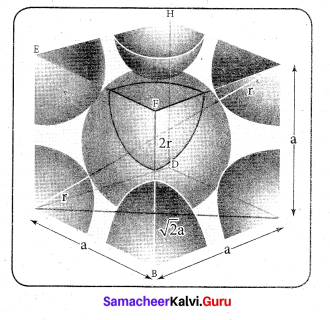Number of spheres belong to a unit cell in bec arrangement is equal to two and hence the total volume of all spheres.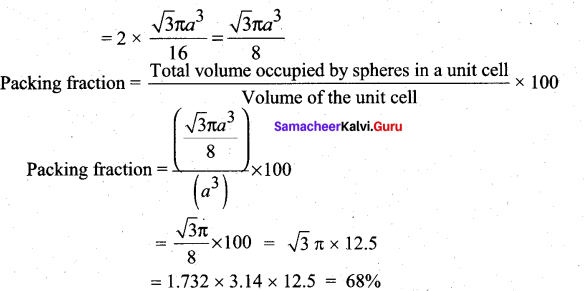i. e., 68% of the available volume is occupied.The available space is used more efficiently than in simple cubic packing.Question 28.
H3BO3 accepts hydroxide ion from water as shown below
H3BO3 (aq) + H2O((l) ⇌ B(OH)4 + H+
Predict the nature of H3BO3 using Lewis concept.
Boric acid is also called as hydrogen borate or orthoboric acid. It is a weak mono basic Lewis acid of boron and it is written as B(OH)3 It accepts hydroxyl (OH) ion from water. It does not dissociate to give hydronium (H3O+) ion rather forms metaborate ion and this ions in turn give H3O+ ion.
B(OH)3 + H2O ⇌ [B(OH)4] + H3O+
Hence it is considered as weak acid.

Question 29.
(ii) When amount of adsorption is plotted versus temperature at constant pressure is called adsorption isobar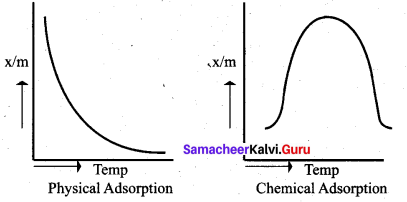(iii) In physical adsorption x/m decreases with increase in T. But in chemical adsorption x/m increases with rise in temperature and then decreases. The increase illustrate the requirement of activation of the surface for adsorption is due to the fact that formation of activated complex require certain energy. The decrease at high temperature is due to desorption, as the kinetic energy of the adsorbate increases.

Question 30.
Mention the uses of Glycerol.

• Glycerol is used as a sweetening agent in confectionery and beverages.
• It is used in the manufacture of cosmetics and transparent soaps.
• It is used in making printing inks and stamp pad ink and lubricant for watches and clocks.
• It is used in the manufacture of explosive like dynamite and cordite by mixing it with china clay.

Question 31.
Give the differences between primary and secondary structure of proteins.
Primary structure of proteins:

• Linear sequence of amino acids
• Composed of peptide bonds formed between amino acids.
• Formed during translation.
• Involved in post – translational modifications.

Secondary structure of proteins :

• Folding of the peptide chain into an a-helix and p-sheet.
• Encompasses hydrogen bonds
• Forms collagen, elastin action, myosin, and keratin – like fibres.
• Involved in forming structures such as cartilages, ligaments, skins etc.

Question 32.
Draw the structure of (i) procaine (ii) Lidocaine
(i) Procaine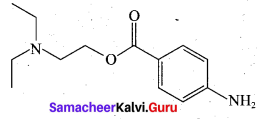(ii) Lidocaine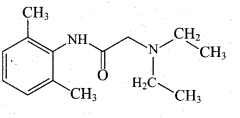Question 33.
Explain the variation in E0M3+/M2+ 3d series.
1. In transition series, as we move down from Ti to Zn, the standard reduction potential $$\mathbf{E}^{0} \mathrm{M}^{2+} / \mathrm{M}^{3+}$$ value is approaching towards less negative value and copper has a positive reduction potential, i.e. elemental copper is more stable than Cu2+ .

2. $$\mathrm{E}^{0} \mathrm{m}^{2+} / \mathrm{M}$$ value for manganese and zinc are more negative than regular trend. It is due to extra stability arises due to the half filled d5 configuration in Mn2+ and completely filled d10 configuration in Zn2+.

3. The standard electrode potential for the M3+ / M2+ half cell gives the relative stability between M3+ and M2+.

4. The high reduction potential of Mn3+ / Mn2+ indicates Mn2+ is more stable than Mn3+.

5. For Fe3+ /Fe2+ the reductionpotential is 0.77V, and this low value indicates that both Fe3+ and Fe2+ can exist under normal condition.

6. Mn3+ has a 3d4 configuration while that of Mn2+ is 3d5. The extra stability associated with a half filled d sub-shell makes the reduction of Mn very feasible [E0 = +1.51 V]

Part – IV

Answer all the questions. [5 x 5 = 25]

Question 34.
(a) (i) Describe a method for refining nickel. (3)
(ii) Why group 18 elements are called inert gases?-Write the general electronic configuration of group 18 elements. (2)
[OR]
(b) (i) Give the uses of helium. (3)
(ii) Ni (II) compounds are more stable than Pt (II) compounds. Give reason. (2)
(a) (i) The impure nickel is heated in a stream of carbon monoxide at around 350 K. The nickel
reacts with the CO to form a highly volatile nickel tetracarbonyl. The solid impurities ‘ . are left behind.
Ni (s) + 4 CO (g) → Ni(CO)4 (g)
On heating the nickel tetracarbonyl around 460 K, the complex decomposes to give pure metal
Ni(CO)4(g) → Ni(s) + 4 CO (g)

(ii) The elements of group-18 have completely filled s and p orbitals, hence they are more stable and have least reactivity. Therefore group-18 elements are called inert gases. ns2np6 is the general electronic configuration of group 18 elements.

[OR]

(b) (i) Uses of helium.

• Helium and oxygen mixture is used by divers in place of air oxygen mixture. This prevents the painful dangerous condition called bends.
• Helium is used to provide inert atmosphere in electric arc welding of metals
• Helium has lowest boiling point hence used in cryogenics (low temperature science).
• It is much less denser than air and hence used for filling air balloons

(ii) The ionisation enthalpy values can be used to predict the thermodynamic stability of their compounds.
For Nickel, I.E1 + I.E2 = 737 + 1753
= 2490 kJ mol-1
For Platinum, I.E1 +I.E2 = 864+ 1791
= 2655 kJ mol-1
Since, the energy required to form Ni2+ is less than that of Pt2+ , Ni(II) compounds are thermodynamically more stable than Pt(II) compounds.

Question 35.
(a) (i) Arrange the following in order of increasing molar conductivity (3)
1. Mg[Cr(NH3)(Cl)5] . 2.[Cr(NH3)5Cl]3 [COF6]2 3. [Cr(NH3)3Cl3]
(ii) Silicon carbide is very hard. Justify this statement. (2)
[OR]
(b) (i) Write the rate law for the following reactions: (a) A reaction that is 3/2 order in x and zero order in y. (A) A reaction that is second order in NO and first order in Br2. (3)
(ii) Classify the following species into Lewis acids and Lewis bases and show how these can act as Lewis acid / Lewis base? (2)
(a) OHions (A) F (c) H+ (d) BCl3
(a) (i) These complexes can ionise in solution as:
Mg[Cr(NH3)(Cl)5] = Mg2+ + [Cr(NH3)(Cl)5]2-
[Cr(NH3 )5Cl]3 [COF6]2 = [Cr(NH3)56Cl]2+ + [COF6]3-
[Cr(NH3)3Cl3] = does not ionize

As the number of ions in solution increases, their molar conductivity also increases.
Therefore, conductivity follows the order:
[Cr(NH3)3Cl3] < [Cr(NH3)5Cl]3 [COF < Mg[Cr(NH3)(Cl)5]

(ii) Silicon carbide is very hard. It is a covalent solid contains the atoms which are bound together in a three dimensional network entirely by covalent bonds. So the covalent network crystal siC is very hard and have high melting point.

[OR]

(b) (i)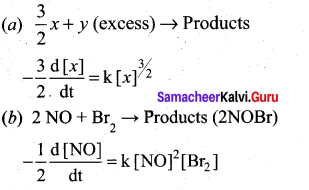(ii) (a) OH ions can donate an electron pair and act as Lewis base.
(b) F ions can donate an electron pair and act as Lewis base.
(c) H+ ions can accept an electron pair and act as Lewis base. .
(d) BCl3 can accept an electron pair since Boron atom is electron deficient. It is a Lewis acid.

Question 36.
(a) (z) Calculate pH of 10-7 MHCl. (3)
(ii) Define corrosion. Give one example. (2)
[OR]
(a) (z) If we do not consider [H3O+ ] from the ionisation of H2O, then [H3O+ ] = [HCl] = 10-7 M i.e., pH = 7, which is a pH of a neutral solution. We know that HCl solution is acidic whatever may be the concentration of HCl i.e, the pH value should be less than 7. In this case the concentration of the acid is very low (10-7 M). Hence, the H3O+ (10-7 M) formed due to the auto ionisation of water cannot be neglected.
So, in this case we should consider [H3O+ ] from ionisation of H2 O
[H3O+ ] = 10-7 (from HCl) + 10-7 (from water)
= 10-7 (1+1)
= 2 x 10-7
pH = -log10[H3O+]
= log10 (2 x 10-7 )=—[log 2 + log10-7 ]
= – log 2 -(-7). 1og1010
= 7 – log 2
= 7 – 0.3010 = 6.6990
= 6.70

(ii) The redox process which causes the deterioration of metal is called corrosion.
Rusting of iron is an example of corrosion. It is an electro chemical process.

[OR]

(b) i. Adsorption isotherms represents the variation of adsorption at constant temperature. Adsorption isotherm can be studied quantitatively. ii. A plot between the amount of adsorbate adsorbed and pressure or concentration of adsorbate at constant temperature is called adsorption isotherms.

According to Freundlich $$\frac{\mathbf{x}}{\mathrm{m}}=\mathrm{k} \mathrm{P}^{1 / \mathrm{n}}$$
Where x is the amount of adsorbate (or) adsorbed on ‘m’ gm of adsorbent at a pressure of R k and n are constants. Value of ‘n’ is always less than unity.

iv. This equation is applicable for adsorption of gases on solid surfaces. The Same equation becomes $$\frac{\mathbf{x}}{\mathrm{m}}=\mathrm{k} \mathrm{P}^{1 / \mathrm{n}}$$ kc when used for adsorption in solutions with ‘c’ as concentration.

v. These equation quantitatively predict the effect of pressure (or concentration) on the adsorption of gases (or adsorbates) at constant temperature.

vi. Taking log on both sides of equation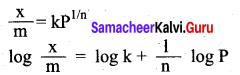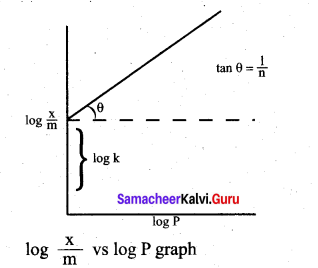vii. Hence the intercept represents the value of log k and the slope $$\frac{b}{q}$$ gives $$\frac{1}{n}$$

viii. This equation explains the increase of $$\frac{x}{m}$$with increase in pressure. But experimental values shows the deviation at low pressure.

ix. Limitations:
(a) This equation is purely empirical and valid over a limited pressure range.
(b) The value of k and n also found vary with temperatures. No theoretical explanations were given.

Question 37.
(a) An organic compound (A) of molecular formula C6H6O gives white precipitate with bromine water. (A) on reaction with NaOH gives (B). (B) reacts with methyl iodide in presence of dry ether gives (C) of molecular formula C7H/8O which will not liberate H2 gas with metallic Na. (C) on reaction with acetyl chloride gives (D) and (E) of formula which are position isomers. Identify A, B, C, D & E and explain the reaction. (5)

[OR]

(A) (i) What happens when n-propyl benzene is oxidised using H+ / KMnO4?(2)
(ii) Identify A, B ,and C.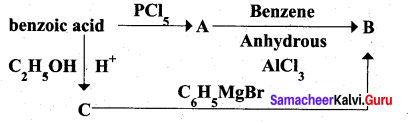1. An organic compound gives white precipitate with bromine water means it must be a phenol. From the molecular formula it is identified as C6H5OH.

2. Phenol on reaction with NaOH gives (B) as sodium phenoxide C6H5ONa.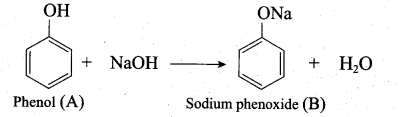3. Sodium phenoxide on reaction with methyl iodide in the pressure of dry ether undergo Williamsons synthesis and gives Anisole as (C).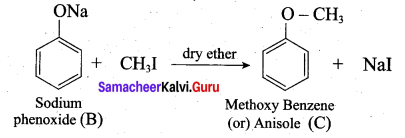4. Anisole on reaction with acetyl chloride undergoes Friedel Craft’s acetylation and yield o-methoxy acetophenone and p-methoxy acetophenone as (D) and (E).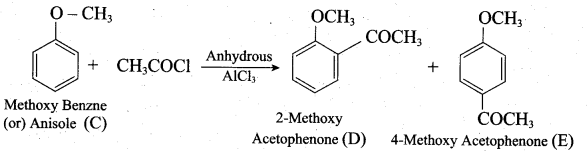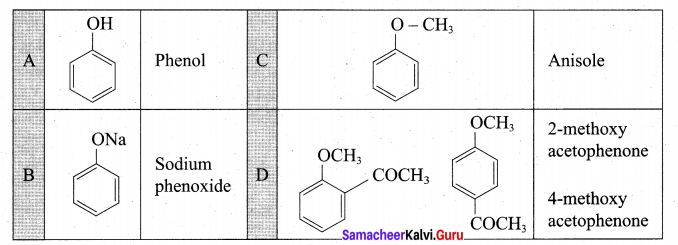(b)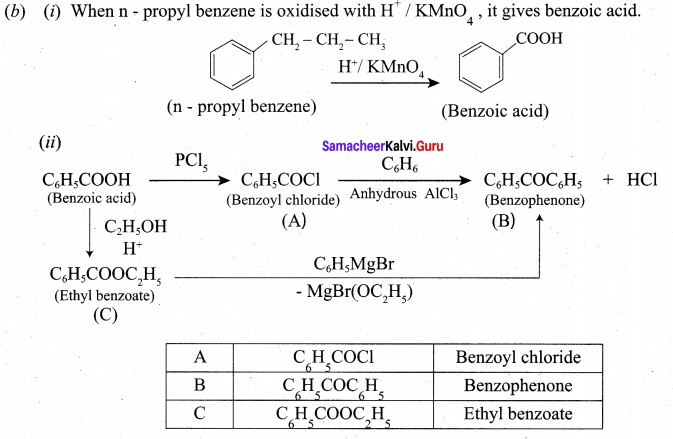Question 38.
(a) (i) How will you distinguish between primary secondary and tertiary alphatic amines. (3)
(ii) Convert Benzene diazonium chloride into phenol. (2)
[OR]
(b) (i) What are the functions of lipids in living organism? (3)
(ii) What is Orion? Give its preparation and use. (2)